Transcripted Summary

So far, the loops we've reviewed have been condition controlled.

There's one more loop, the For Loop, which is count-controlled meaning it loops a given number of times.Let's learn about the For Loop by writing a program for a cashier that will scan a given number of items and tally the cost.

• I created a new class called `Cashier`
• I've already asked the user how many items that they'd like to scan.
• I stored that into this variable `quantity`.
• Also, I made a variable called `total`, and this will hold our total price.
``````package chapter4;

import java.util.Scanner;

/*
* FOR LOOP:
* Write a cashier program that will scan a given number of items and tally the cost.
*/

public class Cashier {

public static void main(String args[]){

//Get number of items to scan
System.out.println("Enter the number of items you would you like to scan:");
Scanner scanner = new Scanner(System.in);

int quantity = scanner.nextInt();

double total = 0;

//Create loop to iterate through all of the items and accumulate the costs
}
}
``````

What we need to do now is create a loop that's going to iterate however many times they told us to. It's going to get the price of each item and then accumulate that price into this total variable.

That's where a for loop comes in. Again, the for loop is count-controlled, meaning it iterates a certain number of times. You write the word `for`, and then in parentheses you're going to have three small statements.

The very first statement of the `for` loop initializes a sentinel, so we'll use this as a counter.

``````for(int i=0;
``````

This `i` is our sentinel. It is a common variable name for our incrementer within the `for` loop. Also, when we're using loops in programming, it is common to start with the number 0 as opposed to the number 1. So, 0 is the first one.

**The next statement in our `for` loop is going to be a condition that ends this loop. **

We'll say keep looping while `i` is less than the quantity:

``````for(int i=0; i<quantity;
``````

So, if `i` is starting at 0, and let's say they entered something like 3, then we'll iterate while i is 0, 1, and 2 so that will be three times, the same as the quantity.

Then the final statement is we say `i++`:

So, this final one doesn't take a semicolon. Then we add our curly braces.

``````for(int i=0; i<quantity; i++){

}
``````

The `++` is going to increment `i` by 1, because remember we're using `i` as a counter.

This will keep track of how many times we have iterated through this loop — and iterate just means go through the loop.

Now inside of this loop, what are we going to do?

We're going to ask the user for the cost of the item, and then we're going to input that and we're going to add it to our running total.

So, let me do that part now.

``````for(int i=0; i<quantity; i++){

System.out.println("Enter the cost of the item:");
double price = scanner.nextDouble();

total = total + price;
}
``````

Okay, so we've asked them for the item, we have input this in, and then we are adding it to the total. So, this again (`total`), is called an accumulator where you're basically just continuously adding into another variable.

Once we've gotten the total, we're going to print it out.

Now we're not going to print this out inside of the loop, because this loop is going to execute multiple times and we don't want to keep printing it out — unless we were giving a subtotal after each one. But we're not going to do that, we're just going to print the total at the end.

### # Cashier.java

``````package chapter4;

import java.util.Scanner;

/*
* FOR LOOP:
* Write a cashier program that will scan a given number of items and tally the cost.
*/
public class Cashier {

public static void main(String args[]){

//Get number of items to scan
System.out.println("Enter the number of items you would you like to scan:");
Scanner scanner = new Scanner(System.in);
int quantity = scanner.nextInt();

double total = 0;

//Create loop to iterate through all of the items and accumulate the costs
for(int i=0; i<quantity; i++){

System.out.println("Enter the cost of the item:");
double price = scanner.nextDouble();

total = total + price;
}

scanner.close();

System.out.println("Your total is \$" + total);
}
}
``````

All right, so let's go ahead and run it.

It goes inside of the loop. We’ll say to run 3 times and enter 20, 30, and 40 as the costs of the items.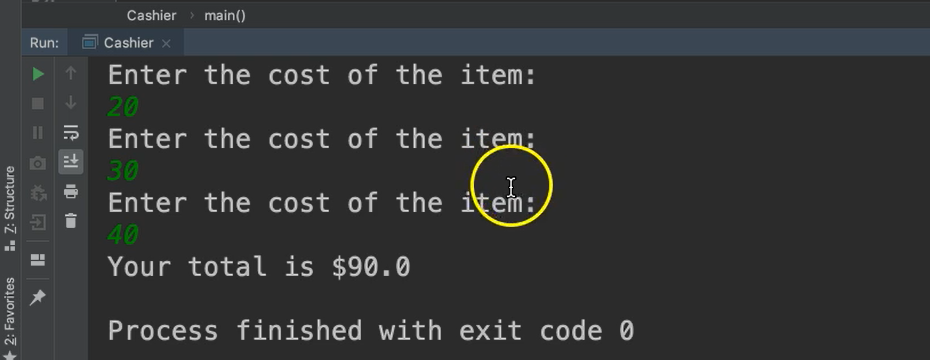Then it comes outside of the loop and prints 90.

So, we knew how many times we wanted to go in. It went and executed exactly those number of times.

### # Key Points of the For Loop

Here are the key points about the `for` loop.• It's count-controlled, meaning it will run a specified number of times.
• The sentinel is expressed within a condition which is tested before the loop is entered.
• It's best to use when you know how many times the loop should be executed.

### # Steps of the For Loop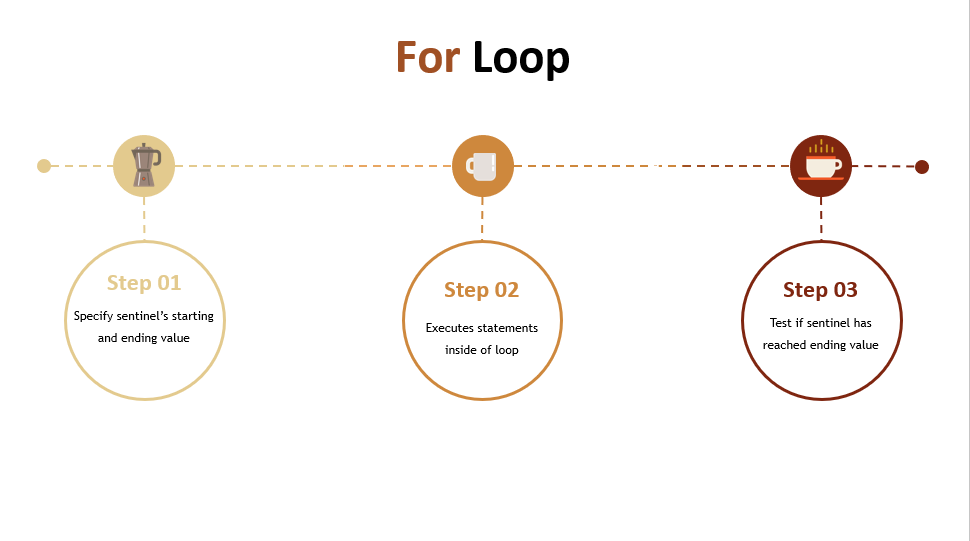1. First, the sentinel is initialized to a starting value and the ending value is also specified.
2. Then the statements inside of the loop or executed
3. And the condition is rechecked to determine if to run the loop again.

Those are the three loops — the `while`, the `do while`, and the `for` loops, and we see how they're controlled.

### # Break Statement

Sometimes you may need to get out of a loop regardless of what the condition is, and we can use the `break` statement for this.

Let's do another example so that I can demonstrate this in action.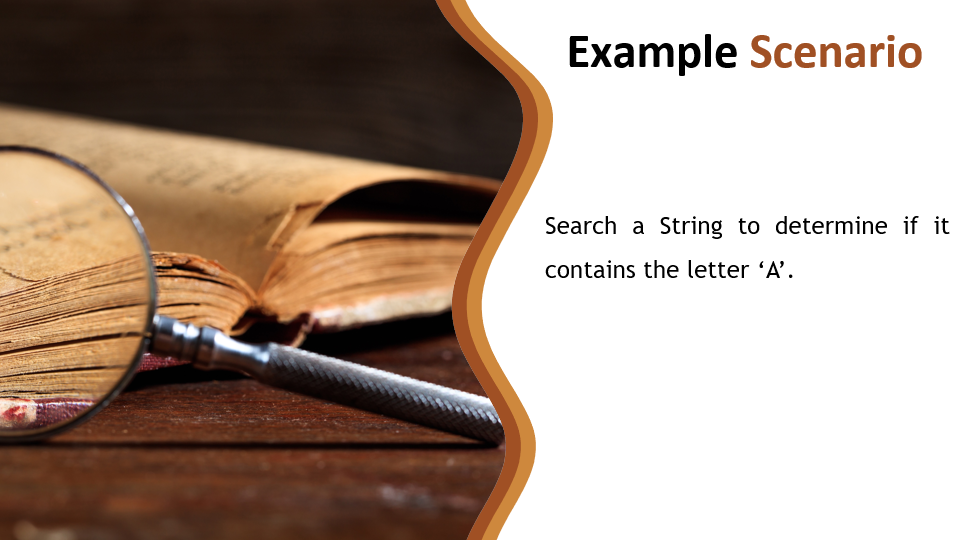We're going to create a program that searches a String to determine if it contains the letter A.

``````package chapter4;

import java.util.Scanner;

/*
* LOOP BREAK
* Search a String to determine if it contains the letter 'A'.
*/
public class LetterSearch {

public static void main(String args[]){

//Get text
System.out.println("Enter some text:");
Scanner scanner = new Scanner(System.in);
String text = scanner.next();
scanner.close();
}
}
``````

So far, in this program, I have prompted the user to enter some text. I have stored that text within a String, and now we're going to loop through that text to determine if it contains the letter A.

The loop that we're going to use is a `for` loop.

We're going to start at `i`, and in this condition we're going to say loop until you reached the end of the String. Now with Strings, they have a nice little method here called `length`. This is the same number of characters that exist in that String. So, we can say keep going until we get to the end of that. And of course, `i++` to increment this.

``````//Search text for letter A
for(int i=0; i<text.length(); i++){

}
``````

Now inside of this `for` loop, I'm going to get the letter that we're currently on, so in the first iteration of this loop, we're going to be on the very first letter.

I can set that to a `char` and we'll call this `currentLetter`

``````//Search text for letter A
for(int i=0; i<text.length(); i++){
char currentLetter = text.charAt(i);
}
``````

Every time we come through this loop, `i` will be incremented — so the first time it gets the very first letter, the second time the second letter, 3rd, 4th and so on.

Once we have whatever letter we're working with, we can check to see if that letter equals A.

Or it might be a lowercase ‘a’, so we'll add that condition as well. If it's A, then we can update a variable called `letterFound`.

``````boolean letterFound = false;
//Search text for letter A
for(int i=0; i<text.length(); i++){
char currentLetter = text.charAt(i);
if(currentLetter == 'A' || currentLetter == 'a'){
letterFound = true;
}
}
``````

Then here's the key.

Once I found that this letter is true — let's say that they've entered this long text of 100 characters, and the second letter was an A —there's no reason I should still let this loop continue until the very end, because I already know that it contains A.

So, to be efficient, we want to get out of this loop once we find it, so we can use this keyword `break`.

Breaking Out of a Loop

`break` will end the loop. It doesn't matter what iteration it's on.

So, if we're on the second iteration and we find it, we're going to come outside of this loop, and we're going to continue on with the program.

At this point, let's go ahead and let them know if we found the letter.

### # LetterSearch.java

``````package chapter4;

import java.util.Scanner;

/*
* LOOP BREAK
* Search a String to determine if it contains the letter 'A'.
*/
public class LetterSearch {

public static void main(String args[]){

//Get text
System.out.println("Enter some text:");
Scanner scanner = new Scanner(System.in);
String text = scanner.next();
scanner.close();

boolean letterFound = false;

//Search text for letter A
for(int i=0; i<text.length(); i++){
char currentLetter = text.charAt(i);
if(currentLetter == 'A' || currentLetter == 'a'){
letterFound = true;
break;
}
}

if(letterFound){
System.out.println("This text contains the letter 'A'");
}
else{
System.out.println("This text does not contain the letter 'A'");
}
}
}
``````

Okay, great, let's run it — but I'm going to use a debugger.

### # Debugging

In your code editor, there's usually a debugger. With IntelliJ, there's definitely one. You can use it to stop at any point within your execution of your program. I want to stop it right after we get what the `currentLetter` is.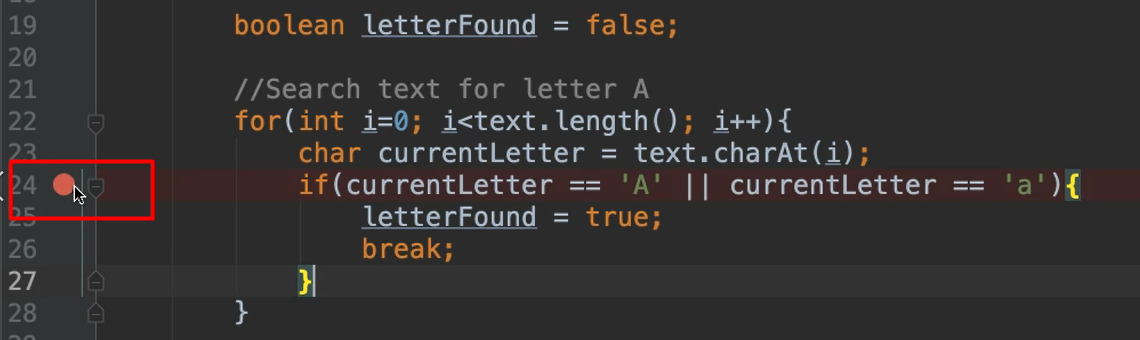So, at this line, I'm simply going to click it and that adds what's called a breakpoint.

When your program is executing, once it gets to the breakpoint, it'll stop and give you information about everything so far.

To run this in debug mode, instead of clicking the play button, you're going click this “bug” icon, which is debug.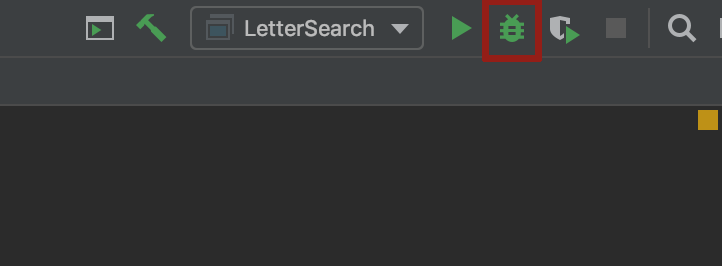I'm going to run this, and it's asking for some text.

Let's enter “Beach”, where A is the 3rd letter.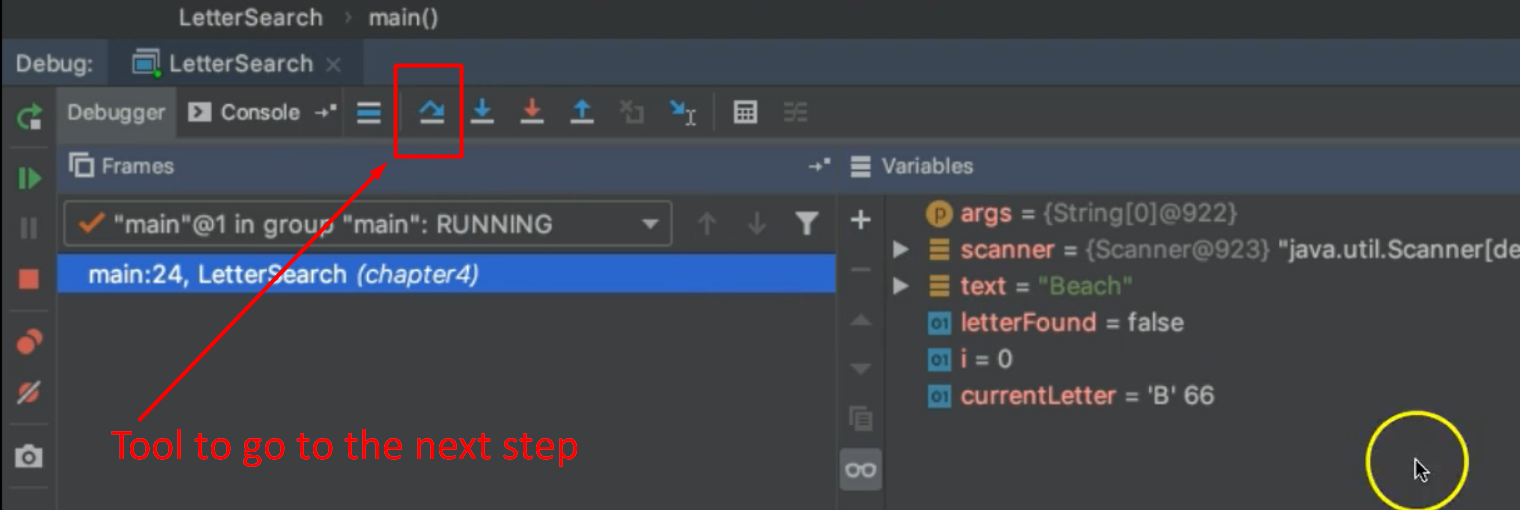Now the program is executing, and it stopped at the breakpoint. Notice right here it's given us all of this information.

• The text is “Beach”
• The `letterFound` is false
• `i` is currently on 0. meaning the first letter
• The `currentLetter` we're looking at is “B”

Now to continue through this program, we can use these little tools right here. This is saying “go to the next step”.

• So, we're going to evaluate that `if`statement. The condition is false, so it came back to the top of this `for` loop.
• And now we see that it's updating `i`, and then the `currentLetter` is now “e”. Now we're on the `if` statement. This is also going to be false, so it's going to go back to the top of the loop.
• Now we see that the `currentLetter` is “a”. Now it's going to evaluate this condition. Notice it's true, so it went inside of this `if` block. We update `letterFound`to true.
• Now we're going to break outside of the loop, never going back in for the fourth letter.

Now we're outside of our `for` loop, and we don't need to debug anymore. We just want to run it all. We can click this to see resume.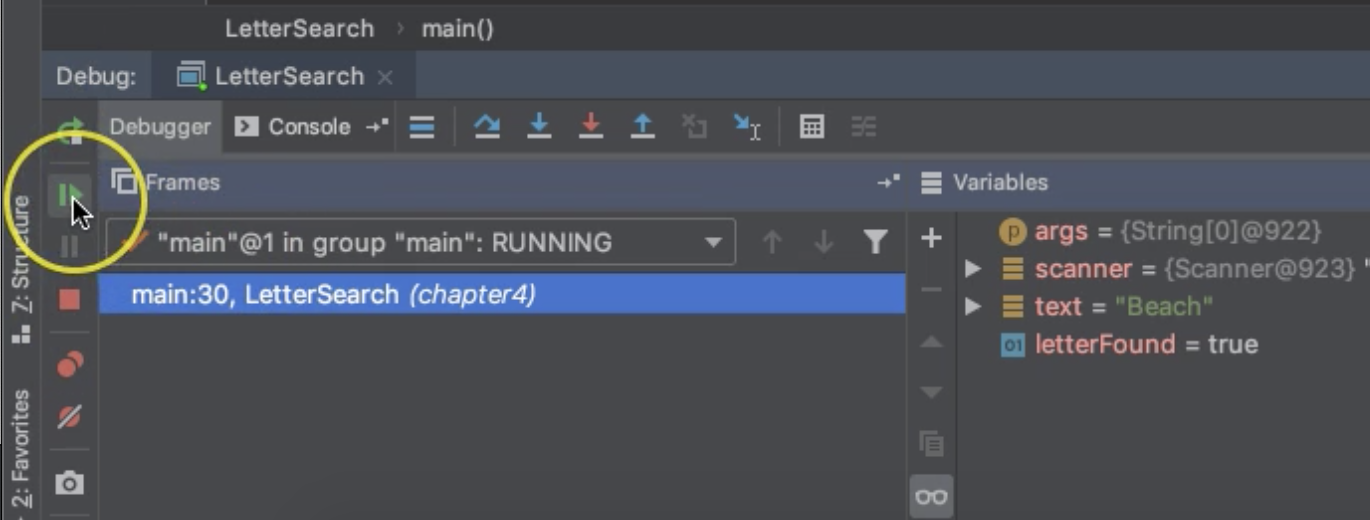And then back to the console here, and it says yep, this text contains the letter A.

## Resources

Quiz

The quiz for Chapter 4 can be found at the end of Section 4d